# Little hand

What angle shifted little hand on the clock after one hour and 38 minutes?

x =  49 °

### Step-by-step explanation: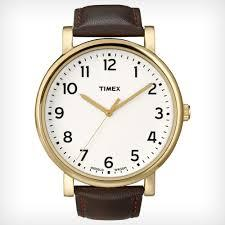Did you find an error or inaccuracy? Feel free to write us. Thank you!Tips to related online calculators
Do you want to convert time units like minutes to seconds?

## Related math problems and questions:

• Clock hands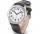The hands-on clock shows the time 12 hours and 2 minutes. Calculate the size of an acute angle between clock hands three hours later.
• ClocksHow will long path walk the end of the clock's minute-hand long 7 cm for 330 minutes? How long will walk in the same time hour-hand?
• Clock Tower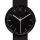What angle is betwenn hands on Clock Tower when show 17 hours and 35 minutes?
• HandsThe clock shows 12 hours. After how many minutes will angle between the hour and minute hand 90°? Consider the continuous movement of both hands hours.
• Speed of clockIn how many minutes will the hour hand pass a straight angle and a right angle?
• Minute-hand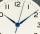How long distance will travel a large (minute) hand on the clock for 48 minutes, if its length is 56 cm?
• Minute handWhat is the distance the minute hand of the clock travels in 12 minutes, if the diameter of the clock is 30 cm and the hand extends to a distance of 2 cm from the edge of the clock?
• Time clockWhat are the angle of the hour hand and the minute hand if it is 0:40?
• Clock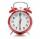What distance will pass end of 8 cm long hour hand for 15 minutes?
• Clock mathematicsIf it is now 7:38 pm, what time will it be in 30,033,996,480 minutes from now?
• Motion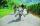Cyclist started at 9:00 from point S to point T. After 10 minutes, followed him at the same speed the second cyclist. Walker, which went from T to S, started at 9:35. After 71 minutes he met the first cyclist and after next 8 minutes the second cyclist. D
• ClocksHow long track travel the second hand of a clock in 46 hours, which is 4 cm long.
• Compass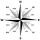What angle are between directions WSW (WestSouthWest) and SSE (SouthSouthEast) on the compass?
• BusesBuses Ikarus and Karosa simultaneously started at 8:00 from the final station. Ikarus is returned to the station after 30 minutes. Karosa after 45 minutes. At what time both buses again returned to the station?
• Clock handsCalculate the internal angles of a triangle whose vertices lie on the clock's 2, 6 and 11 hours.
• Clock losing time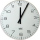A clock is showing 9:30 am. If the clock is 5 minutes slow, what would have been the correct time?
• Clocks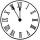What distance will describe the tip of minute hand 6 cm long for 20 minutes when we know the starting position with finally enclose hands each other 120°?